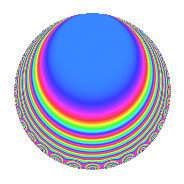# Properties

 Label 3136.1.dLevel 3136 Weight 1 Character orbit d Rep. character $$\chi_{3136}(1471,\cdot)$$ Character field $$\Q$$ Dimension 7 Newform subspaces 4 Sturm bound 448 Trace bound 5

# Related objects

## Defining parameters

 Level: $$N$$ $$=$$ $$3136 = 2^{6} \cdot 7^{2}$$ Weight: $$k$$ $$=$$ $$1$$ Character orbit: $$[\chi]$$ $$=$$ 3136.d (of order $$2$$ and degree $$1$$) Character conductor: $$\operatorname{cond}(\chi)$$ $$=$$ $$4$$ Character field: $$\Q$$ Newform subspaces: $$4$$ Sturm bound: $$448$$ Trace bound: $$5$$

## Dimensions

The following table gives the dimensions of various subspaces of $$M_{1}(3136, [\chi])$$.

Total New Old
Modular forms 74 12 62
Cusp forms 26 7 19
Eisenstein series 48 5 43

The following table gives the dimensions of subspaces with specified projective image type.

$$D_n$$ $$A_4$$ $$S_4$$ $$A_5$$
Dimension 3 4 0 0

## Trace form

 $$7q + 3q^{9} + O(q^{10})$$ $$7q + 3q^{9} + q^{25} + 2q^{29} + 6q^{37} + 6q^{53} - 4q^{57} - 4q^{65} - q^{81} - 4q^{93} + O(q^{100})$$

## Decomposition of $$S_{1}^{\mathrm{new}}(3136, [\chi])$$ into newform subspaces

Label Dim. $$A$$ Field Image CM RM Traces $q$-expansion
$$a_2$$ $$a_3$$ $$a_5$$ $$a_7$$
3136.1.d.a $$1$$ $$1.565$$ $$\Q$$ $$D_{2}$$ $$\Q(\sqrt{-1})$$, $$\Q(\sqrt{-7})$$ $$\Q(\sqrt{7})$$ $$0$$ $$0$$ $$0$$ $$0$$ $$q+q^{9}-q^{25}+2q^{29}+2q^{37}-2q^{53}+\cdots$$
3136.1.d.b $$2$$ $$1.565$$ $$\Q(\sqrt{-1})$$ $$A_{4}$$ None None $$0$$ $$0$$ $$-2$$ $$0$$ $$q+iq^{3}-q^{5}-iq^{11}-iq^{15}+q^{17}+\cdots$$
3136.1.d.c $$2$$ $$1.565$$ $$\Q(\sqrt{2})$$ $$D_{4}$$ $$\Q(\sqrt{-1})$$ None $$0$$ $$0$$ $$0$$ $$0$$ $$q-\beta q^{5}+q^{9}+\beta q^{13}-\beta q^{17}+q^{25}+\cdots$$
3136.1.d.d $$2$$ $$1.565$$ $$\Q(\sqrt{-1})$$ $$A_{4}$$ None None $$0$$ $$0$$ $$2$$ $$0$$ $$q-iq^{3}+q^{5}-iq^{11}-iq^{15}-q^{17}+\cdots$$

## Decomposition of $$S_{1}^{\mathrm{old}}(3136, [\chi])$$ into lower level spaces

$$S_{1}^{\mathrm{old}}(3136, [\chi]) \cong$$ $$S_{1}^{\mathrm{new}}(196, [\chi])$$$$^{\oplus 5}$$$$\oplus$$$$S_{1}^{\mathrm{new}}(784, [\chi])$$$$^{\oplus 3}$$$$\oplus$$$$S_{1}^{\mathrm{new}}(1568, [\chi])$$$$^{\oplus 2}$$

## Hecke characteristic polynomials

$p$ $F_p(T)$
$2$ 1
$3$ ($$( 1 - T )( 1 + T )$$)($$1 - T^{2} + T^{4}$$)($$( 1 - T )^{2}( 1 + T )^{2}$$)($$1 - T^{2} + T^{4}$$)
$5$ ($$1 + T^{2}$$)($$( 1 + T + T^{2} )^{2}$$)($$1 + T^{4}$$)($$( 1 - T + T^{2} )^{2}$$)
$7$ 1
$11$ ($$( 1 - T )( 1 + T )$$)($$1 - T^{2} + T^{4}$$)($$( 1 - T )^{2}( 1 + T )^{2}$$)($$1 - T^{2} + T^{4}$$)
$13$ ($$1 + T^{2}$$)($$( 1 + T^{2} )^{2}$$)($$1 + T^{4}$$)($$( 1 + T^{2} )^{2}$$)
$17$ ($$1 + T^{2}$$)($$( 1 - T + T^{2} )^{2}$$)($$1 + T^{4}$$)($$( 1 + T + T^{2} )^{2}$$)
$19$ ($$( 1 - T )( 1 + T )$$)($$1 - T^{2} + T^{4}$$)($$( 1 - T )^{2}( 1 + T )^{2}$$)($$1 - T^{2} + T^{4}$$)
$23$ ($$( 1 - T )( 1 + T )$$)($$1 - T^{2} + T^{4}$$)($$( 1 - T )^{2}( 1 + T )^{2}$$)($$1 - T^{2} + T^{4}$$)
$29$ ($$( 1 - T )^{2}$$)($$( 1 + T^{2} )^{2}$$)($$( 1 + T^{2} )^{2}$$)($$( 1 + T^{2} )^{2}$$)
$31$ ($$( 1 - T )( 1 + T )$$)($$1 - T^{2} + T^{4}$$)($$( 1 - T )^{2}( 1 + T )^{2}$$)($$1 - T^{2} + T^{4}$$)
$37$ ($$( 1 - T )^{2}$$)($$( 1 - T + T^{2} )^{2}$$)($$( 1 + T^{2} )^{2}$$)($$( 1 - T + T^{2} )^{2}$$)
$41$ ($$1 + T^{2}$$)($$( 1 + T^{2} )^{2}$$)($$1 + T^{4}$$)($$( 1 + T^{2} )^{2}$$)
$43$ ($$( 1 - T )( 1 + T )$$)($$( 1 - T )^{2}( 1 + T )^{2}$$)($$( 1 - T )^{2}( 1 + T )^{2}$$)($$( 1 - T )^{2}( 1 + T )^{2}$$)
$47$ ($$( 1 - T )( 1 + T )$$)($$1 - T^{2} + T^{4}$$)($$( 1 - T )^{2}( 1 + T )^{2}$$)($$1 - T^{2} + T^{4}$$)
$53$ ($$( 1 + T )^{2}$$)($$( 1 - T + T^{2} )^{2}$$)($$( 1 - T )^{4}$$)($$( 1 - T + T^{2} )^{2}$$)
$59$ ($$( 1 - T )( 1 + T )$$)($$1 - T^{2} + T^{4}$$)($$( 1 - T )^{2}( 1 + T )^{2}$$)($$1 - T^{2} + T^{4}$$)
$61$ ($$1 + T^{2}$$)($$( 1 - T + T^{2} )^{2}$$)($$1 + T^{4}$$)($$( 1 + T + T^{2} )^{2}$$)
$67$ ($$( 1 - T )( 1 + T )$$)($$1 - T^{2} + T^{4}$$)($$( 1 - T )^{2}( 1 + T )^{2}$$)($$1 - T^{2} + T^{4}$$)
$71$ ($$( 1 - T )( 1 + T )$$)($$( 1 - T )^{2}( 1 + T )^{2}$$)($$( 1 - T )^{2}( 1 + T )^{2}$$)($$( 1 - T )^{2}( 1 + T )^{2}$$)
$73$ ($$1 + T^{2}$$)($$( 1 + T + T^{2} )^{2}$$)($$1 + T^{4}$$)($$( 1 - T + T^{2} )^{2}$$)
$79$ ($$( 1 - T )( 1 + T )$$)($$1 - T^{2} + T^{4}$$)($$( 1 - T )^{2}( 1 + T )^{2}$$)($$1 - T^{2} + T^{4}$$)
$83$ ($$( 1 - T )( 1 + T )$$)($$( 1 - T )^{2}( 1 + T )^{2}$$)($$( 1 - T )^{2}( 1 + T )^{2}$$)($$( 1 - T )^{2}( 1 + T )^{2}$$)
$89$ ($$1 + T^{2}$$)($$( 1 + T + T^{2} )^{2}$$)($$1 + T^{4}$$)($$( 1 - T + T^{2} )^{2}$$)
$97$ ($$1 + T^{2}$$)($$( 1 + T^{2} )^{2}$$)($$1 + T^{4}$$)($$( 1 + T^{2} )^{2}$$)